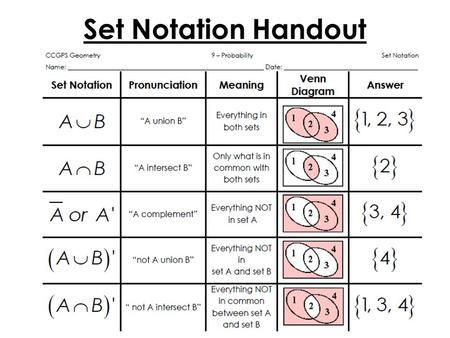# Math background

## Sets

Set a collection of distinct elements:

• ${a, b, c, d}$ - finite
• $\mathbb{R}$ : real numbers - infinite
• ${x \in \mathbb{R}: cos(x) > 1/2}$ - set with restriction

Notations:

• $\Omega$ - universal set(set that contain all objects)
• $\in$ - is member of
• $2 \in \{1, 2, 3, 4\}$
• $\notin$ - is not member of
• $2 \notin \{1, 3, 5\}$
• $A’$, $A^c$, $\overline{\rm A}$ - $A$ compliment, everything not in $A$
• $x \in S^c if x \in \Omega, x \notin S$
• $\emptyset$ and $\varnothing$ or $\{ \}$ - empty set
• $\Omega^c = \emptyset$
• $\cup$ - union, everything in both sets
• $\{1, 2, 3\} \cup \{3, 4, 5\} = \{1, 2, 3, 4, 5\}$
• $x \in S \cup T \leftrightarrow x \in S \, \textsf{or} \> x \in T$
• $\cup_{n} S_{n}$ - union of all $n$ sets
• $\cap$ - intersection, only what is in common in both sets
• $\{1, 2, 3\} \cap \{2, 3, 4\} = \{2, 3\}$
• $x \in S \cap T \leftrightarrow x \in S \, \textsf{and} \, x \in T$
• $\cap_{n} S_{n}$ - intersection of all $n$ sets
• $\subset$ - subset of set
• $\{1, 2, 3\} \subset \{1, 2, 3, 4, 8\}$
• $\supset$ - superset of set
• $\{1, 2, 3, 4, 8\} \supset \{1, 2, 3\}$### Sets properties

• $S \cup T = T \cup S$
• $S \cap (T \cup U) = (S \cap T) \cup (S \cap U)$
• $(S^c)^c = S$
• $S \cup \Omega = \Omega$
• $S \cap S^c = \emptyset$
• $S \cap \Omega = S$
• $S \cup (T \cup U) = (S \cup T) \cup U$
• $S \cup (T \cap U) = (S \cup T) \cap (S \cup U)$

### De Morgan’s laws

• $(S \cap T)^c = S^c \cup T^c$
• $(\cap_{n} S_{n}^c) = \cup_{n} S_{n}^c$
• $(S \cup T)^c = S^c \cap T^c$
• $(\cup_n S_{n}^c) = \cap_n S_{n}^c$

### Countable versus uncountable infinite sets

Countable: can be put in 1-1 correspondence with positive integers:

• Positive integers
• Integers: $0, 1, -1, 2, -2, …$
• Pairs of positive integers
• Rational numbers $q$, with $0 < q < 1$, $1/2, 1/3, 2/3, 1/4, 2/4, …$

Uncountable: not countable:

• The interval [0, 1]
• The reals
• The plane

## Sequences and their limits

Sequence is some collection of an elements:

• $a_1, a_2, a_3, …$
• sequence $a_1$
• $\{a_i\}$
• formal - function $f: \mathbb{N} -> S, f(i) = a_i$

When does a sequence converge:

• If $a_i \leq a_{i + 1}$ for all $i$, then either:
• the sequence “converges to $\infty$
• the sequence converges to some real number $a$
• if $|a_i - a| \leq b_i$ for all $i$, and $b_i -> 0$, then $a_i -> a$

## Series

### Infinite series

$\sum_{i=1}^{\infty} a_i = \lim_{n -> \infty} \sum_{i=1}^n a_i$ (provided limit exists)

• if $a_i \geq 0$: limit exists
• if terms $a_i$ do not all have the same sign:
• limit need not exist
• limit may exist but be different if we sum in different order
• Fact: limit exists and independent of order of summation if $\sum_{i=1}^{\infty} |a_i| < \infty$

### Order of summation in series

only if $\sum |a_{ij}| < \infty$, then:

• $\sum_{i \geq 1, j \geq 1} a_{ij} = \sum_{i=1}^{\infty}(\sum_{j = 1}^{\infty} a_{ij}) = \sum_{j=1}^{\infty}(\sum_{i = 1}^{\infty} a_{ij})$
• $\sum_{(i, j) : j \leq i} a_{ij} = \sum_{i=1}^{\infty} \sum_{j=1}^{i} a_{ij} = \sum_{j=1}^{\infty} \sum_{i=j}^{\infty} a_{ij}$

### Arithmetic progression

\begin{equation*} a_{n}=a_{1}+(n-1)d \end{equation*}
\begin{equation*} a_{n}=a_{m}+(n-m)d \end{equation*}
\begin{equation*} S_n = \frac{n}{2}[2a_1 + (n - 1)d] \end{equation*}
\begin{equation*} S_n = \frac{n}{2}[a_1 + a_n] \end{equation*}
\begin{equation*} \overline{\rm n} = S_n / n \end{equation*}
\begin{equation*} \overline{\rm n} = \frac{a_1 + a_n}{2} \end{equation*}

where:

• $a_1$ is the first term of an arithmetic progression.
• $a_n$ is the nth term of an arithmetic progression.
• $d$ is the difference between terms of the arithmetic progression.
• $n$ is the number of terms in the arithmetic progression.
• $S_n$ is the sum of n terms in the arithmetic progression.
• $\overline{\rm n}$ is the mean value of arithmetic series.

### Geometric progression

\begin{equation*} a_n = ar^{n - 1} \end{equation*}
\begin{equation*} a_n = ra_{n - 1} \end{equation*}
\begin{equation*} S_n = \frac{a_1 (1 - r^n)}{1 - r} \end{equation*}
\begin{equation*} S_n = \frac{a_1 - a_n * r}{1 - r} \end{equation*}
\begin{equation*} where r \ne 1 \end{equation*}

where:

• $a$ - initial value
• $r$ - common ratio

Simplified: $S = \sum_{i=0}^{\inf} \alpha^i = 1 + \alpha + \alpha^2 + … = \frac{1}{1 - \alpha}$, if $|\alpha| < 1$

pass

## Other

If $X$ is a vector, and $X \in \{-1, 1\}^{n}$, then $X^{T}X == n$, where $n$ is a constant, and $n == len(X)$.